Hey everyone! I hope you're all doing well and having a good time. I'm going to give you a thorough overview of MC34063 IC. It's usually required when building DC to DC Boost or Buck converters. We will learn how to create a 5V to 12V Boost Converter Circuit using the MC34063 DC-to-DC Converter IC in this blog.The MC34063 is a DC-DC converter IC that's commonly used in Buck (step-down) and Boost (step-up) circuits. The MC34063 is a monolithic control circuit that includes all of the functionality needed to build DC to DC converters. It can switch at a frequency of up to 100 kHz. It's employed in DC-to-DC converters because of its voltage conversion ability. It works in a temperature range of 0 to 70 degrees Celsius. It's used in voltage regulators and chargers, among other things. An 8-pin DIP package contains all of these functions.

MC34063 IC can perform multiple functions:

• Output voltage-sense comparator
• Oscillator.
• High current output switch.
• Active Peak Current Limit
• MC 34063 requires minimum externals equipment for the buck, boost applications.
• It has a lot of applications including, cable solutions, blood gas analyzer, telecommunications, etc
• Temperature-compensated reference voltage

PINOUT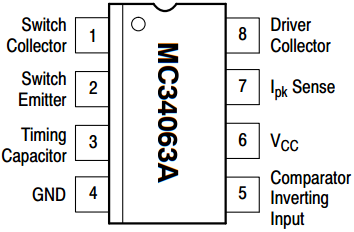Pin Number Pin Name Description 1 Switch Collector Collector pin of Internal transistors (Output Voltage pin) 2 Switch Emitter Emitter pin of Internal Transistors 3 Timing Capacitor Connect to a capacitor which decides the switching frequency 4 Ground Negative Terminal of power supply 5 Comparator Inverting Input Used to set the output voltage 6 VCC Input Voltage 7 Ipeak Sense Used to set the output current 8 Driver Collector Collector pin of switching transistor

The IC MC34063 has a wide input supply range of 3V to 40V and may generate a high output switch current of up to 1.5A with the addition of a suitable inductor. It offers adjustable output voltage, short circuit current limiting, and a low standby current. We can include this IC into the buck, boost, and inverting topologies by utilising a few extra components.

DC-DC converters are frequently used to efficiently provide a regulated voltage from a variable source that may or may not be properly controlled to a variable load. A Buck Converter produces less voltage than the initial voltage, but a Boost Converter produces more voltage.

The switching regulator IC MC34063A is at the core of the circuit. The Schottky diode 1N5819 has a low forward voltage drop and a fast switching speed. It is frequently utilized in high-frequency applications such as inverters and DC-DC converters.

Because the MC34063A can handle up to 40V, the circuit can handle DC voltages ranging from 3.0V to 40.0V. It offers adjustable output voltage, short circuit current limiting, and a low standby current.

Basic Calculation of a Boost Converter(5VIN, 12VOUT)

Now at this step we will start building our circuit and the component which will be present in it and of course their values.

Here is the schematic without the values of the components, which will be used additionally with the MC34063.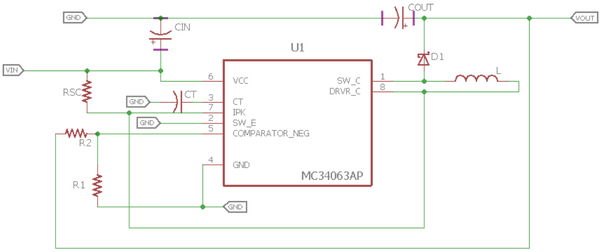If we check the datasheet, we can see the complete formula chart present to calculate the desired values required as per our requirement. Here is the formula sheet available inside the datasheet, and as per our requirement, we have the step-up circuit formulas.

Now we will calculate the values which are required for our design. We can make the calculations from the formulas provided in the datasheet.

Steps to calculate those components valuesStep 1:-

For the calculations first, we need to select a Diode. We will choose widely available diode 1N5819. So as per the datasheet, at 1A forward current the forward voltage of the diode will be 0.49 V.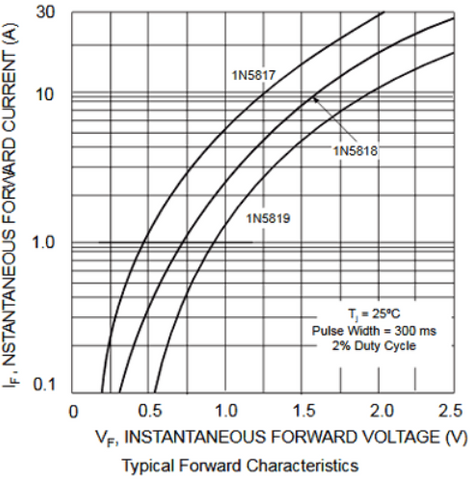Step 2:- We will calculate Ton/Toff using the formula

For this, our Vout is 12V, Forward voltage of the diode (Vf) is 0.49V. Our minimum Voltage Vin (min) is 4.5V. And for the saturation voltage of the output switch (Vsat), is 0.45V (0.45V in the datasheet). By, putting this all together in the formula.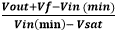(12+0.49-4.5 )/(4.5-0.45) = 1.97

So, Ton / Toff = 1.97

Step 3:- No we will calculate the Ton + Toff time, as per the formula:

Ton + Toff = 1 / f

The switching frequency will be 50Khz,as per the datasheet.

So, Ton + Toff = 1 / 50Khz =  20us

So the Ton + Toff will be 20uS

Step 4:-For the next step we will calculate the Toff time.

Toff = (Ton + Toff / (Ton/Toff )+1)

As per the previous calculations in Step-2 and Step-3, the calculation will be easier now,

Toff = 20us / 1.97+1 =  6.73us

Step 5:- In the next step we will calculate Ton,

We will need to us Step-3 and Step-4 for this calculation

Ton = (Ton + Toff) - Toff = 20us – 6.73us = 13.27s

Step 6:- We will need to choose the timing Capacitor Ct, which will be required to produce the desired frequency.

Ct = 4.0 x 10-5 x Ton , we will take the value of Ton from Step-5

= 4.0 x 10-5 x 13.27uS = 530.8pF(converted from micro farad to pico farad)

A 560pF creamic capacitor is available in the market so the user can buy that.

Step 7:- Now we need to calculate the Peak current or Average inductor current in the circuit

Ipk = 2 x Iout(max) x ((Ton/Toff )+1)             ,Ton/Toff from Step-2

Our maximum output current will be 200mA. So, the Peak current current will be  2 x 0.2 x (1.97+ 1) = 1.2A.

Step 8:- Now we need to calculate the Sense current Resistor Value, the resistor which will be connected to the 7th pin of the IC.

Rsc =  0.3/Ipk                         , Ipk from the Step-7

0.3/1.2 = 0.25ohm

So, the Rsc will be 0.25ohm

Step 9:- Depending on those values we will calculate the Inductor value

Lmin= ( (Vin(min) - Vsat/ Ipk(switch) )  ) x (Ton(max))

= ( 4.5 - 0.45/ 1.2 )  ) x (13.27) =44.8uH  , Ipk from the Step-7, Ton from Step-5

Inductor value = 45uH

Step 10:- So now Let’s calculate the output capacitor’s values

We can choose a ripple value of 200mV (peak to peak) from the boost output.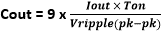So, Cout = 9* (0.2 * 13.27us / 0.2) = 119.43uF

We will use a 120uF 20V Capacitor. The more capacitor will be used the more ripple it will reduce.

Step 11:- Last we need to calculate the feedback voltage resistors value.

Vout = 1.25 (1 + R2 / R1), keeping Vout= 12V

R2=8.6 R1, So this means the R2 will be 8.6 times the R1

We will choose R1 value 910 ohm, So, the R2 value will be 8.2K ohm

We have calculated all the required values so if you wish to use a Boost converter you could take the help from these calculations and build your circuit.

If you wish to buy MC34063 IC they you may click HERE